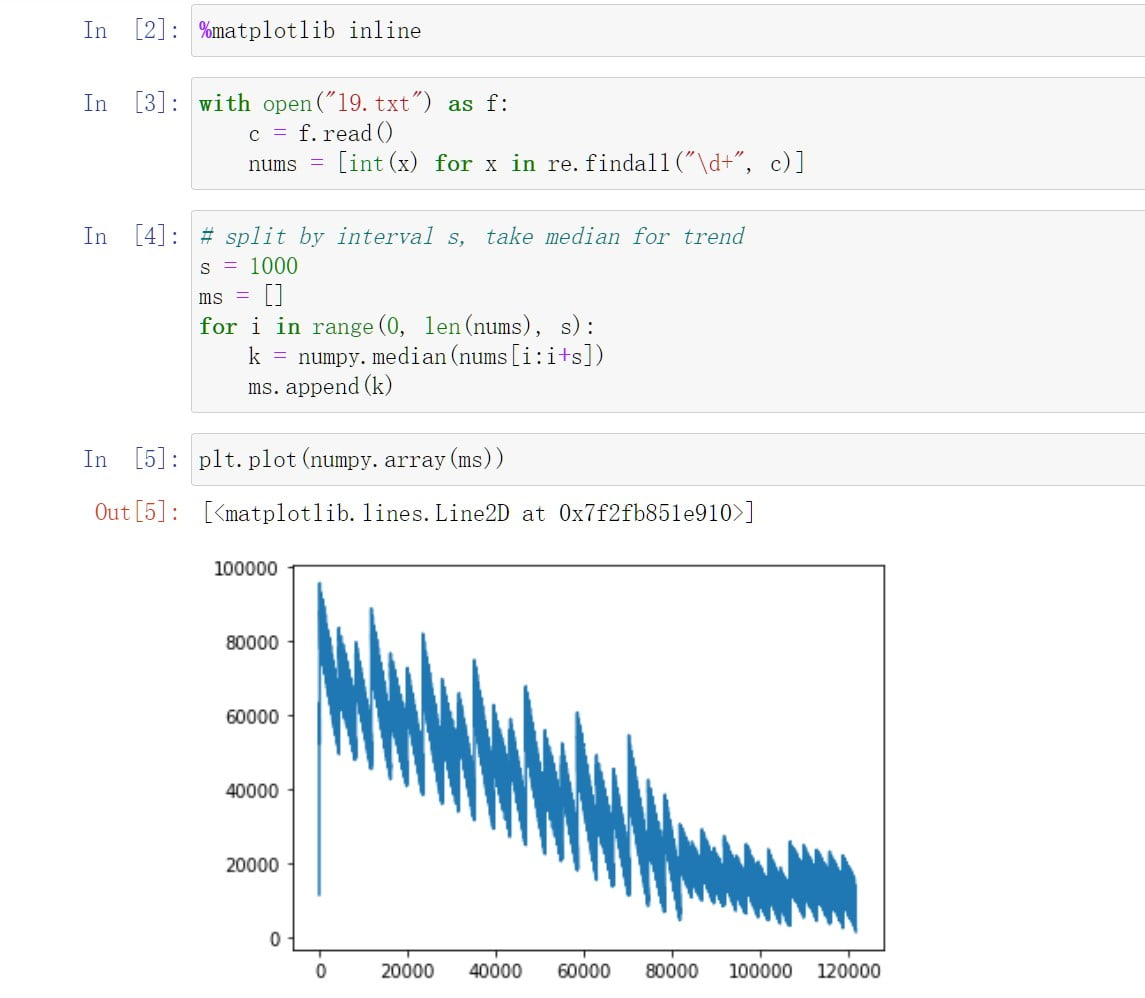Previously, 2015 writeup.

### Day 2

The second map can be encoded into a 5x5 grid, then start from `[-2 0]`, skip steps when going outside `[0 0]` + 2 manhattan distance.

``````(def d2map2 ["00100" "02340" "56789" "0ABC0" "00D00"])
(defn d2-map2? [loc] (> (reduce + (map abs loc)) 2)) ;; true to skip current instruction

;; final string
(apply str
(for [[x y] locs]
(get-in d2map2 [(+ 2 y) (+ 2 x)])))
``````

`get-in` looks cleaner than nested `nth`.

### Day 3

Transpose:

``````(def i3 "  566  477  376
575  488  365
50   18  156
558  673  498")
(let [ls (map #(re-seq #"\d+" %) (clojure.string/split-lines i3))]
(->> ls
(apply mapv vector)
(flatten)
(partition 3)))
=> (("566" "575" "50") ("558" "477" "488") ("18" "673" "376") ("365" "156" "498"))
``````

### Day 6

Transpose again:

``````(let [ti6 (apply mapv vector (clojure.string/split-lines i6))
chs (for [t ti6] ;; remove 'reverse' for part 2
(->> t frequencies (sort-by second) reverse first first))]
(apply str chs))
``````

### Day 9

”..you’ll have to come up with another way to get its decompressed length” if memory is not enough.

Add following to `project.clj`: `:jvm-opts ["-Xmx60g"]`, and recursively expand the string:

• find index `i` of first “(“, return if fully expanded
• else get the substring `s1` to be repeated, substring `s2` right to s1
• check if `s1` contains “(“, recur with `[i k*s1+s2]` or `[i+k*len(s) s2]`
• add accumulated `i` and string left

This, though slow, keeps the length of string to be expanded under 10x of origin input:### Day 11

Generator/Microchip pairs are equivalent, so any state can be represented as `[floor [[p0 s0] [p1 s1] [p2 s2] [p3 s3]]]`, where p0 is pairs count in floor 0, s0 is single component count.

Encoding this way reduces count of costs from 6042584 to 11427, and makes (still naive) BFS much faster: 2 parts in 7 seconds.

source: you can get quite a decent speedup by doing two BFS’s in lockstep. One from the initial state, and one from the goal state. Then when they meetup you add the two distances to get the answer.

### Day 19

Use core.rrb-vector for “faster concatenation of vectors”.

Some encountered bug CRRBV-14, my 2017-17 input triggered this exception too:

``````(defn step-buggy
[v i s]
(let [m (count v) ;; the number to insert, for  it's 1
-i (mod (+ i s) m) ;; idx to be insert-after
ni (inc -i) ;; the new index
[l r] (if (>= ni m)
[v []]
[(fv/subvec v 0 ni) (fv/subvec v ni)])]
[(fv/catvec l [m] r) ni s]))

(let [n 37890 ;; 50000000
[v i s] (reduce (fn [[v i s] _]
(step-buggy v i s))
[ 0 377]
(range n))]
(fv/subvec v 0 10))
``````

### Day 21

Brute force!

``````(for [p (combo/permutations "abcdefgh")
:let [s (apply str p)]
:when (= "fbgdcaeh" (enc21 s))]
s)
``````

### Day 24

Like 2015-9, build distance map then:

• part 1: just concat  with permutations of other digits
• part 2: append 0 to previous path
``````(apply min
(for [p (combo/permutations (remove #(= % 0M) (keys digits)))
:let [path (into [0M] p) ;; concat with start point
path (flatten (conj [0M] p 0M)) ;; part 2: return to 0
rs (partition 2 1 path) ]];; A->B B->C pairs
(reduce + (map dist rs))))
``````